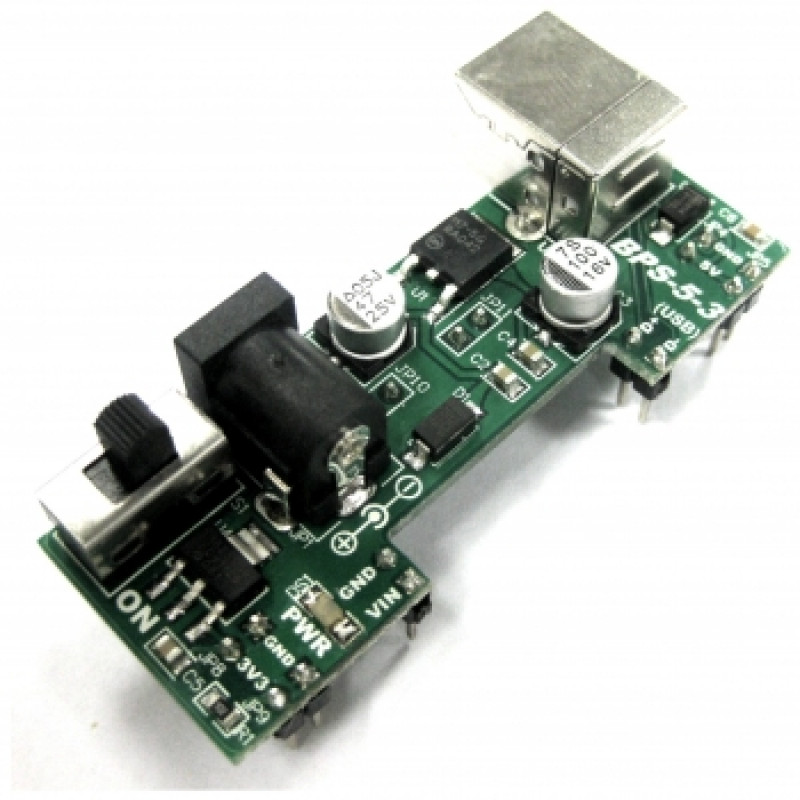# Ultrasonic Range Finder Fast Test!

Introduction

Ultrasonic sensor are easy to be if you are using the Maxbotic Ultrasonic Sensor. It provide various types of output which is already been configure. For this tutorial, I will guide you to use the sensor using normal instrument to see the changes of the sensor.

Hardware required:Project:

Procedure:

First of all, the connection for the ultrasonic sensor is a must. So, lets connect the ultrasonic sensor to the power supply to power it up and test it

ConnectionThis is the look of my connection of the ultrasonic sensor. I know it is a bit complicated but I will display out the easier to view version later on.Here are my connection for the circuit. And we could see the change of the ultrasonic sensor through the multimeter voltage change. Normally it is just like plug in the power of the ultrasonic sensor and tested the ADC output.

connection list

 Connection Ultrasonic Sensor 5V (red) 5V Gnd (black) Gnd multimeter (whilte) ADC

And below the the picture illustrate the result of the change of the ADC signal

Those are the different in voltage when I block and sensor with my hand and one of it is without.

The left side is unblock and the right side is blocked.

The analog voltage pin outputs a voltage which corresponds to the distance. The further away an object is from the sensor the higher the output voltage becomes which in turn will be measured by the multimeter. The sensor is designed to report the range to the closest detectable object.

Calculating the Voltage Scaling

Ultrasonic sensor output is scaled to the input power that is provided to the sensor, it is important to know the voltage scaling before calculating the range.
The formula for the voltage scaling on the ultrasonic sensor EZ1 is:
[(Vcc/512) = Vi]
Vcc = Supplied Voltage
Vi = Volts per inch (Scaling)

Example 1: Say you have an input voltage of +5.0V the formula would read:
[(5.0V/512) = 0.009766V per inch = 9.766mV per inch]

Calculating the Range

Once you know the voltage scaling it is easy to properly calculate the range.
The range formula is:
[(Vm/Vi) = Ri]
Vm = Measured Voltage
Vi = Volts per Inch (Scaling)
Ri = Range in inches

Example 2: To get comfortable with this equation use a known distance by using a ruler. Say the multimeter shows 292.98mV then you use the calculations as follows:
[(292.98mV/9.766mV) = 30 inches]

Example 3: To work backward and verify your calculation is correct use the inverse formula:
[(Ri x Vi) = Vm]
[(30 x 9.766) = 292.98]

Please note that if you are using for the voltage scaling, the scaling will be different. To find the voltage scaling of your sensor reference of your sensor datasheet.
Please note the sensor resolution, for the ultrasonic sensor user-EZ1 the resolution is one inch.

If you have questions or inquires, please do discuss in our technical forum as we seldom check the comments and feedback in tutorial site.

### 2 thoughts on “Ultrasonic Range Finder Fast Test!”

1.what is the maximum range can detect? (maximum voltage as the further the object from the sensor, the higher the output)

2.morning..saya nk tanya tentang penyambungan litar pic dengan ultrasonic SN-LV-EZ1..